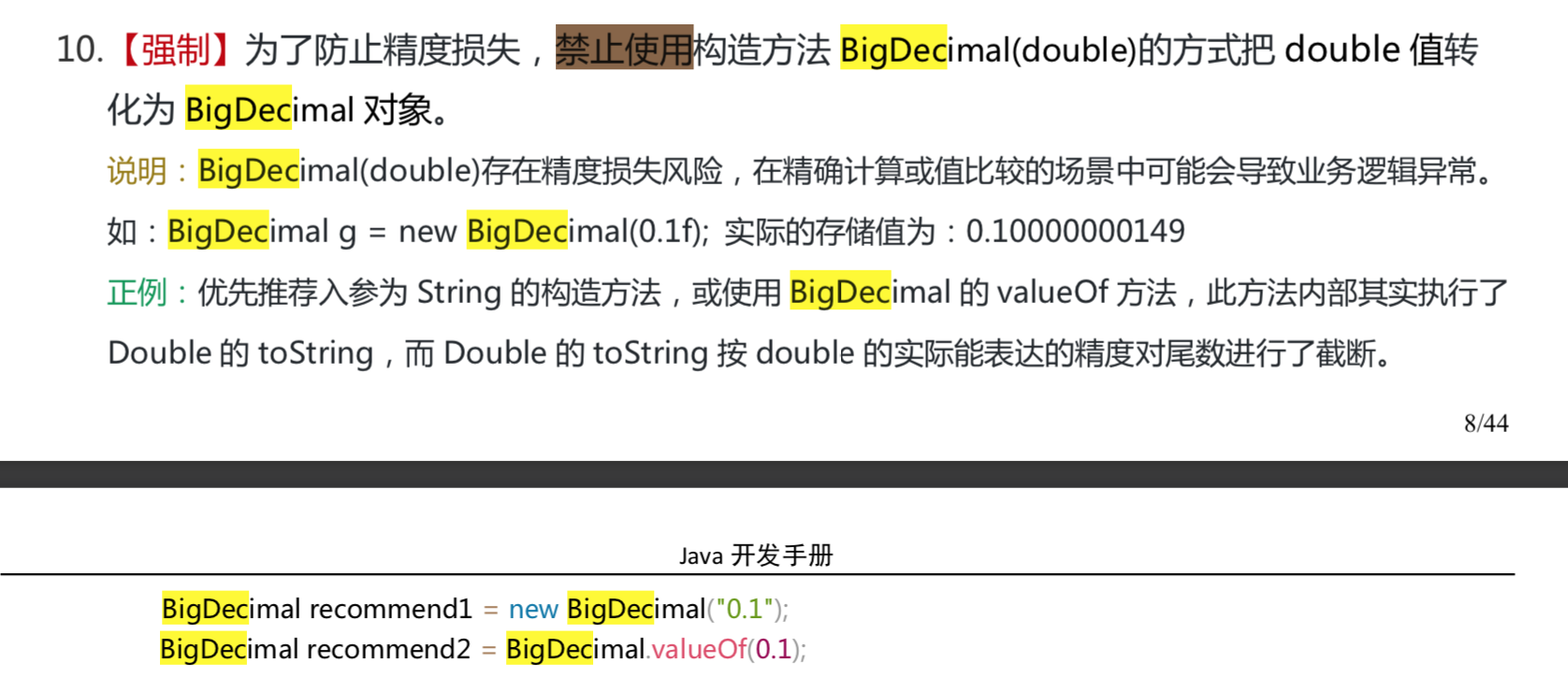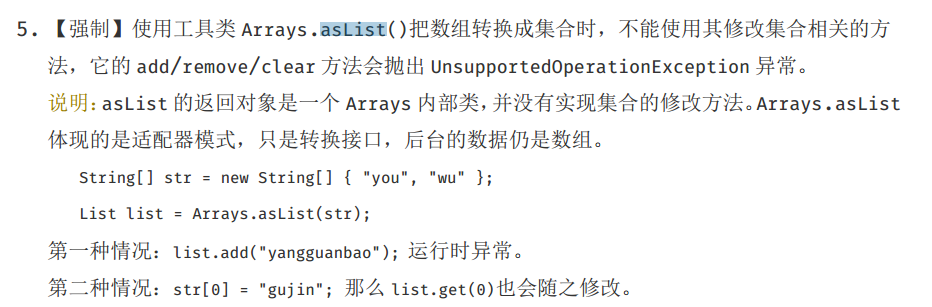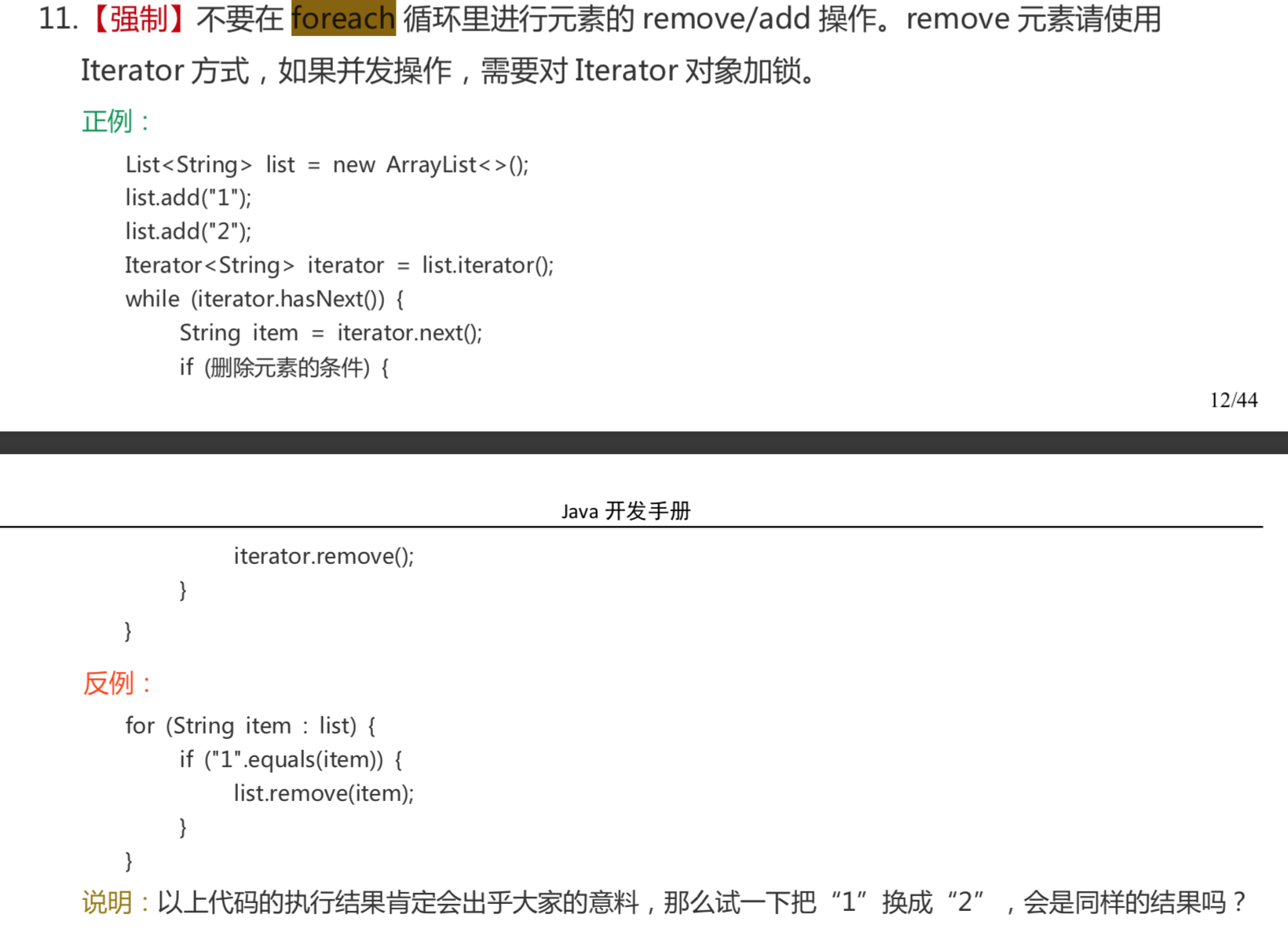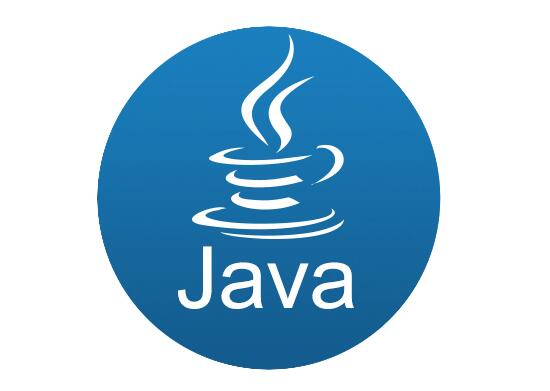# 1. 基础

## 1.1. 正确使用 equals 方法

Object的equals方法容易抛空指针异常，应使用常量或确定有值的对象来调用 equals。

// 不能使用一个值为null的引用类型变量来调用非静态方法，否则会抛出异常

``````String str = null;
if (str.equals("SnailClimb")) {
...
} else {
..
}
``````

``````"SnailClimb".equals(str);// false
``````

``````Objects.equals(null,"SnailClimb");// false
``````

``````public static boolean equals(Object a, Object b) {
// 可以避免空指针异常。如果a==null的话此时a.equals(b)就不会得到执行，避免出现空指针异常。
return (a == b) || (a != null && a.equals(b));
}
``````

Reference:Java中equals方法造成空指针异常的原因及解决方案

1. 每种原始类型都有默认值一样，如int默认值为 0，boolean 的默认值为 false，null 是任何引用类型的默认值，不严格的说是所有 Object 类型的默认值。
2. 可以使用 == 或者 != 操作来比较null值，但是不能使用其他算法或者逻辑操作。在Java中null == null将返回true。
3. 不能使用一个值为null的引用类型变量来调用非静态方法，否则会抛出异常

## 1.2. 整型包装类值的比较

``````Integer x = 3;
Integer y = 3;
System.out.println(x == y);// true
Integer a = new Integer(3);
Integer b = new Integer(3);
System.out.println(a == b);//false
System.out.println(a.equals(b));//true
``````

## 1.3. BigDecimal

### 1.3.1. BigDecimal 的用处

《阿里巴巴Java开发手册》中提到：浮点数之间的等值判断，基本数据类型不能用==来比较，包装数据类型不能用 equals 来判断。 具体原理和浮点数的编码方式有关，这里就不多提了，我们下面直接上实例：

``````float a = 1.0f - 0.9f;
float b = 0.9f - 0.8f;
System.out.println(a);// 0.100000024
System.out.println(b);// 0.099999964
System.out.println(a == b);// false
``````

``````BigDecimal a = new BigDecimal("1.0");
BigDecimal b = new BigDecimal("0.9");
BigDecimal c = new BigDecimal("0.8");
BigDecimal x = a.subtract(b);// 0.1
BigDecimal y = b.subtract(c);// 0.1
System.out.println(x.equals(y));// true
``````

### 1.3.2. BigDecimal 的大小比较

a.compareTo(b) : 返回 -1 表示小于，0 表示 等于， 1表示 大于。

``````BigDecimal a = new BigDecimal("1.0");
BigDecimal b = new BigDecimal("0.9");
System.out.println(a.compareTo(b));// 1
``````

### 1.3.3. BigDecimal 保留几位小数

``````BigDecimal m = new BigDecimal("1.255433");
BigDecimal n = m.setScale(3,BigDecimal.ROUND_HALF_DOWN);
System.out.println(n);// 1.255
``````

### 1.3.4. BigDecimal 的使用注意事项

《阿里巴巴Java开发手册》对这部分BigDecimal的描述### 1.3.5. 总结

BigDecimal 主要用来操作（大）浮点数，BigInteger 主要用来操作大整数（超过 long 类型）。

BigDecimal 的实现利用到了 BigInteger, 所不同的是 BigDecimal 加入了小数位的概念

## 1.4. 基本数据类型与包装数据类型的使用标准

Reference:《阿里巴巴Java开发手册》

• 【强制】所有的 POJO 类属性必须使用包装数据类型。
• 【强制】RPC 方法的返回值和参数必须使用包装数据类型。
• 【推荐】所有的局部变量使用基本数据类型。

# 2. 集合

## 2.1. Arrays.asList()使用指南

### 2.1.1. 简介

Arrays.asList()在平时开发中还是比较常见的，我们可以使用它将一个数组转换为一个List集合。

``````String[] myArray = { "Apple", "Banana", "Orange" }；
List<String> myList = Arrays.asList(myArray);
//上面两个语句等价于下面一条语句
List<String> myList = Arrays.asList("Apple","Banana", "Orange");
``````

JDK 源码对于这个方法的说明：

``````/**
*返回由指定数组支持的固定大小的列表。此方法作为基于数组和基于集合的API之间的桥梁，与Collection.toArray()结合使用。
返回的List是可序列化并实现RandomAccess接口。
*/
public static <T> List<T> asList(T... a) {
return new ArrayList<>(a);
}
``````

### 2.1.2. 《阿里巴巴Java 开发手册》对其的描述

Arrays.asList()将数组转换为集合后,底层其实还是数组，《阿里巴巴Java 开发手册》对于这个方法有如下描述：### 2.1.3. 使用时的注意事项总结

Arrays.asList()是泛型方法，传入的对象必须是对象数组。

``````int[] myArray = { 1, 2, 3 };
List myList = Arrays.asList(myArray);
System.out.println(myList.size());//1
System.out.println(myList.get(0));//数组地址值
System.out.println(myList.get(1));//报错：ArrayIndexOutOfBoundsException
int [] array=(int[]) myList.get(0);
System.out.println(array);//1
``````

``````Integer[] myArray = { 1, 2, 3 };
System.out.println(myList.getClass());//class java.util.Arrays\$ArrayList
``````

private static class ArrayList extends AbstractList
implements RandomAccess, java.io.Serializable
{
...

``````    @Override
public E get(int index) {
...
}

@Override
public E set(int index, E element) {
...
}

@Override
public int indexOf(Object o) {
...
}

@Override
public boolean contains(Object o) {
...
}

@Override
public void forEach(Consumer<? super E> action) {
...
}

@Override
public void replaceAll(UnaryOperator<E> operator) {
...
}

@Override
public void sort(Comparator<? super E> c) {
...
}
}
``````

``````public E remove(int index) {
throw new UnsupportedOperationException();
}
``````

### 2.1.4. 如何正确的将数组转换为ArrayList?

stackoverflow：https://dwz.cn/vcBkTiTW

#### 1. 自己动手实现（教育目的）

``````//JDK1.5+
static <T> List<T> arrayToList(final T[] array) {
final List<T> l = new ArrayList<T>(array.length);

for (final T s : array) {
}
return (l);
}
Integer [] myArray = { 1, 2, 3 };
System.out.println(arrayToList(myArray).getClass());//class java.util.ArrayList
``````

#### 2. 最简便的方法(推荐)

``````List list = new ArrayList<>(Arrays.asList("a", "b", "c"))
``````

#### 3. 使用 Java8 的Stream(推荐)

``````Integer [] myArray = { 1, 2, 3 };
List myList = Arrays.stream(myArray).collect(Collectors.toList());
//基本类型也可以实现转换（依赖boxed的装箱操作）
int [] myArray2 = { 1, 2, 3 };
List myList = Arrays.stream(myArray2).boxed().collect(Collectors.toList());
``````

#### 4. 使用 Guava(推荐)

``````对于不可变集合，你可以使用ImmutableList类及其of()与copyOf()工厂方法：（参数不能为空）

List<String> il = ImmutableList.of("string", "elements");  // from varargs
List<String> il = ImmutableList.copyOf(aStringArray);      // from array

List<String> l1 = Lists.newArrayList(anotherListOrCollection);    // from collection
List<String> l2 = Lists.newArrayList(aStringArray);               // from array
List<String> l3 = Lists.newArrayList("or", "string", "elements"); // from varargs
``````

#### 5. 使用 Apache Commons Collections

``````List<String> list = new ArrayList<String>();
``````

## 2.2. Collection.toArray()方法使用的坑&如何反转数组

``````String [] s= new String[]{
"dog", "lazy", "a", "over", "jumps", "fox", "brown", "quick", "A"
};
List<String> list = Arrays.asList(s);
Collections.reverse(list);
s=list.toArray(new String);//没有指定类型的话会报错
``````

## 2.3. 不要在 foreach 循环里进行元素的 remove/add 操作

fail-fast 机制 ：多个线程对 fail-fast 集合进行修改的时，可能会抛出ConcurrentModificationException，单线程下也会出现这种情况，上面已经提到过。

java.util包下面的所有的集合类都是fail-fast的，而java.util.concurrent包下面的所有的类都是fail-safe的。##### 热门推荐
•浏览(484)
•浏览(479)
•浏览(332)
•浏览(318)
•浏览(293)
•浏览(254)
•浏览(246)
•浏览(229)
•浏览(220)
•浏览(207)